# Beginners Guide to Excel Formulas and Mathematical Operators Using Excel 2007 and 2010

Robbie mostly writes about Skyrim but also occasionally sheds light on the oddities of Microsoft applications such as Excel and Outlook.

Today I am going to look at the basics of using formulas in Excel. Excel is an immensely powerful application which can seem daunting to people who are new to it. In today’s article:

• First, we will look at how to create formulas.
• We will look at using basic mathematical operators + - * / % < > ( ) as well as some more advanced operators & and \$.
• Finally, we will use learn how to use three very useful functions in formulas, SUM, AVERAGE and COUNT.

## Creating Basic Formulas

In order for Excel to treat what you are typing into to a cell as a formula, it needs to start with an equals sign (=). There are two easy ways to let Excel know that you are creating a formula.

• The first is to simply type = after you click on a cell.
• The second is to click on the function bar and type your formula (still starting with a = straight into the bar itself.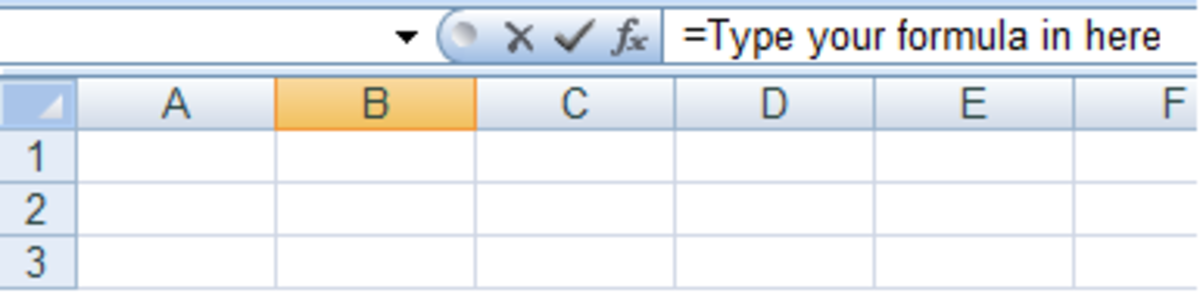How to start entering a formula in Excel 2007 and Excel 2010.http://robbiecwilson.hubpages.com/

Excel 2007 and 2010 have added a very useful tool to help find the best function for your requirements. It is called the Function Library and it allows you to search for functions based on what you need Excel to do. So for example, if you search for “biggest number”, Excel will recommend that you use the MAX function. It will then assist you in creating the formula. You can learn more about how to use this very useful tool here.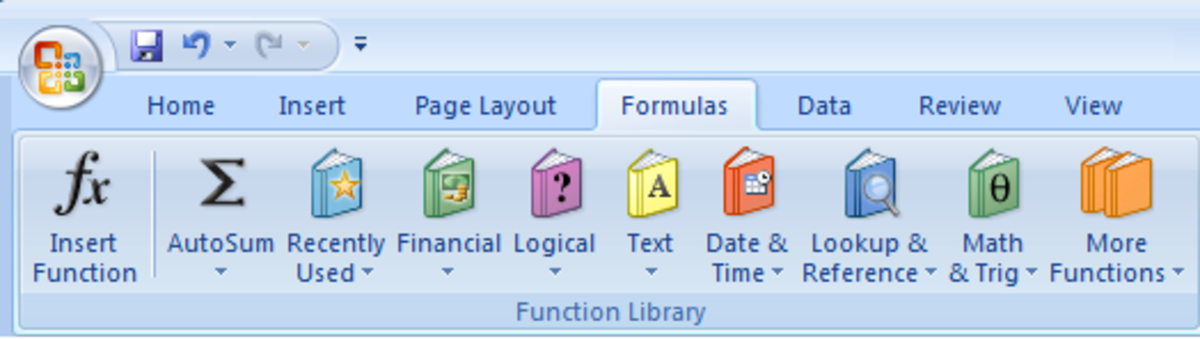The Function Library in Excel 2007 and Excel 2010 allows you to easily select a function for a formula.http://robbiecwilson.hubpages.com/

## Using Mathematical Operators in Formulas

The first formulas that we will create today use the basic mathematical operators + - * / % < > ( )

+ - * / are very easy to use:

• To add 10 and 20 enter =10+20 into a cell
• To subtract 5 from 10 use =10-5
• For multiplying =10*10
• And finally dividing = 10/10

Note: It is important to remember that Excel will perform multiplication and division first before performing subtraction and addition, so =4+2*3 = 10 rather than 18 which you would get if you perform the addition first.

• Percentage is slightly more complicated. Suppose we want to know what percentage 10 is of 20. We would use:

=10/20%

• Using < or > along with = allows you to determine if one number is bigger (or smaller) than another. You would typically use this with a cell’s contents rather than a number. Excel returns TRUE or FALSE for the result. So for example:
Scroll to Continue

=10>=5 returns the result TRUE as 10 is greater than or equal to 5

• The next operator we will look at are the brackets ( ). You use brackets to tell Excel that you want it to calculate one part of the formula before the rest of it.

Using our previous example of =4+2*3

If we want Excel to add 4+2 before multiplying it by 3, our formula becomes =(4+2)*3, the answer of which is 18.

## Using & and \$ in an Excel Formula

The first expression we will look at is the &. This allows you to join the contents of two cells together.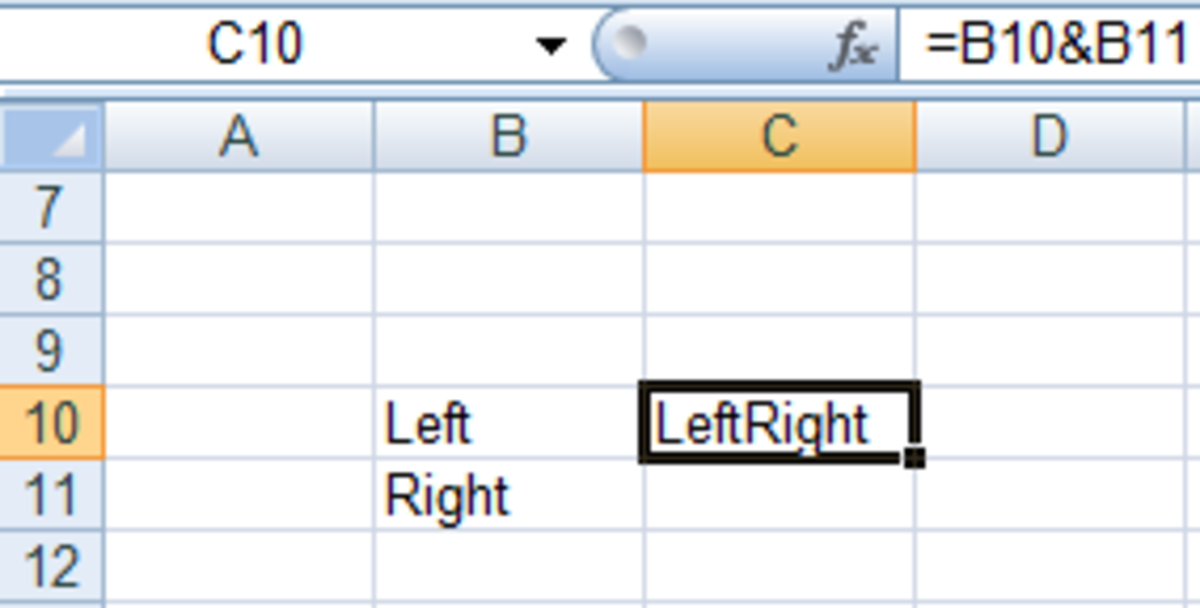Using the & operator in formulas in Excel 2007 and Excel 2010.http://robbiecwilson.hubpages.com/

So =B10&BB11 results in LeftRight. To add a space between Left and Right, use the following syntax

=B10&" "&B11

This gives us the result Left Right.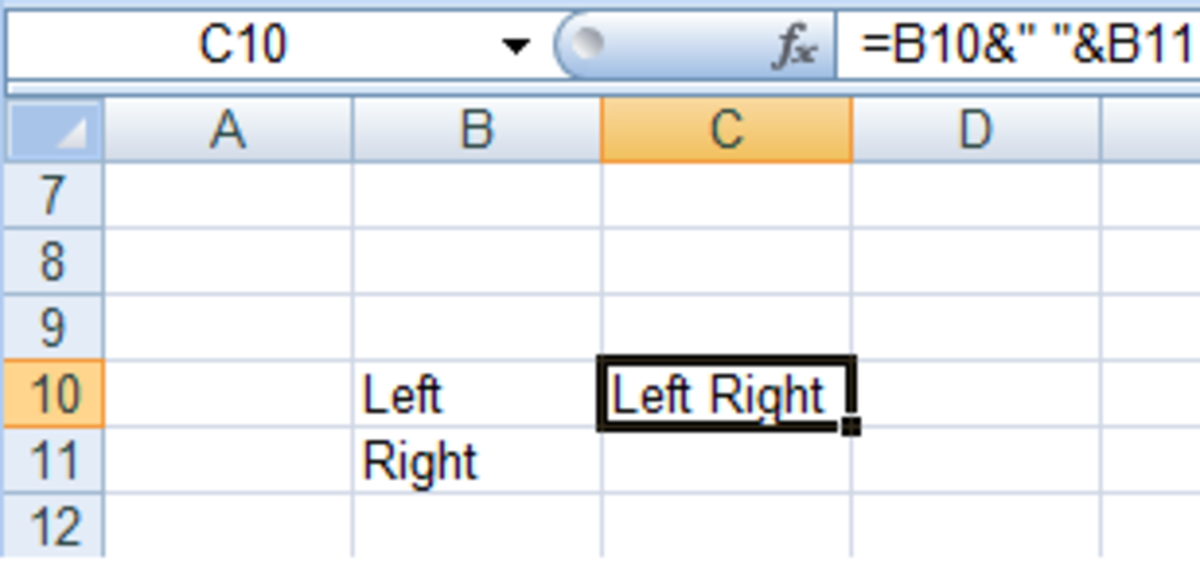Using the & operator and adding spaces in a formula in Excel 2007 and Excel 2010.http://robbiecwilson.hubpages.com/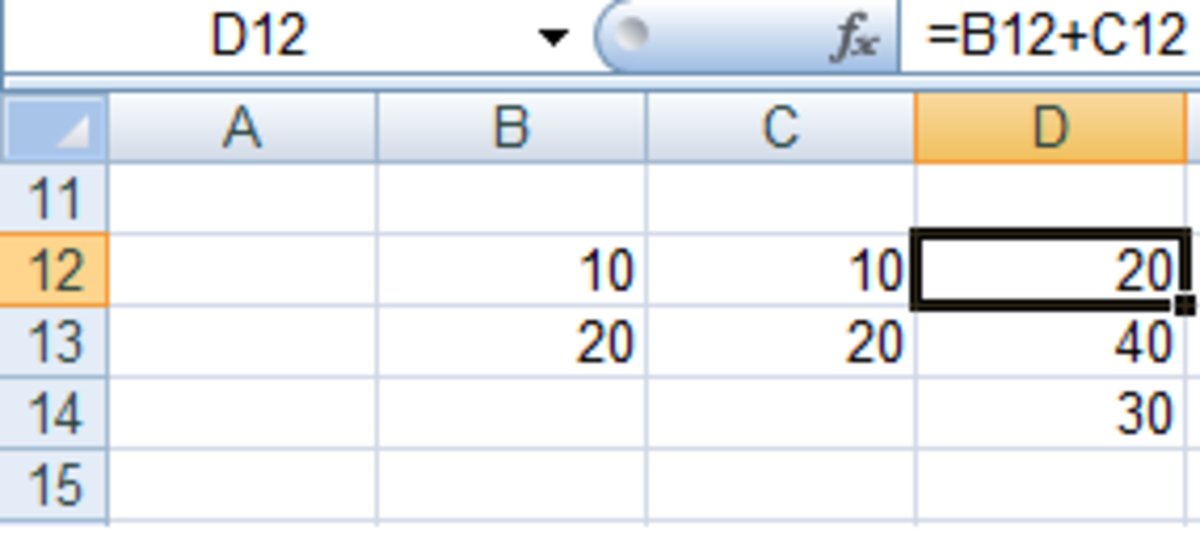An Excel formula without using \$ to change a cell to an absolute reference in Excel 2007 and Excel 2010.http://robbiecwilson.hubpages.com/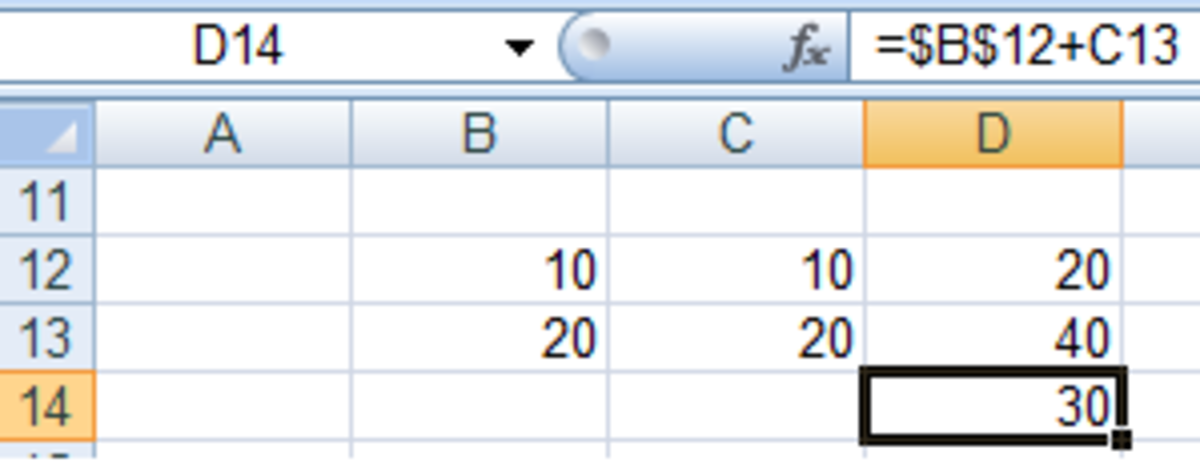Using \$ to change a cell to an absolute reference in a formula in Excel 2007 and Excel 2010.http://robbiecwilson.hubpages.com/

The \$ symbol tells Excel that the cell reference in the formula is an absolute reference and will not change when you copy it.

Note: You need to put a \$ in front of B to fix the column and \$ in front of 12 to fix the row. So \$B13 fixes the column, B\$13 fixes the row and \$B\$13 fixes both.

Let’s illustrate this with an example.

We have a formula =B12+C12 in cell D12.

If we copy the cell containing the formula to D13, the formula becomes =B13+C13.

Suppose you want each formula to add B12 to the contents of the cell in row C.

First change the formula to =\$B\$12+C12 so that Excel always uses B12 in each formula when you copy this formula to another cell.

Now when we copy the formula, B12 stays the same each time and the cell used by Excel in column C changes as before.

## Using the SUM, AVERAGE, and COUNT Function in Formulas

Now that we know the basics of creating formulas and using mathematical operators, we will look at using three of the most useful functions in Excel. These are SUM, AVERAGE and COUNT.

To start, let’s look at the SUM function. You could use + to add up a list of cells, e.g. =A1+A2+A3+A4+A5, but the SUM function allows you to do this quickly and easily:

• To start, type in =SUM(
• Drag the cursor around the cell range you want Excel to sum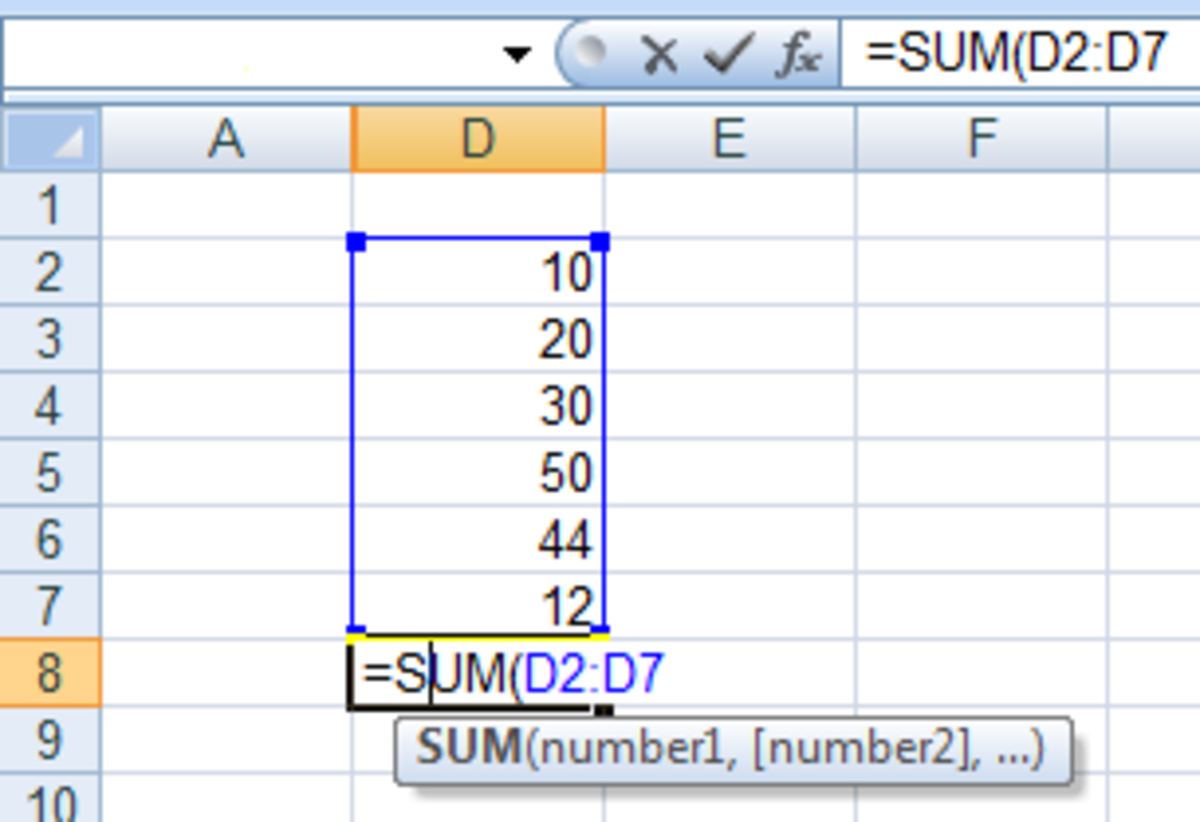How to use the SUM function in a formula in Excel 2007 and Excel 2010.http://robbiecwilson.hubpages.com/
• Add a closing bracket ) and press enter

Excel will add the formula =SUM(D2:D7) into the cell which will give us the answer 166.

AVERAGE works in the same way as SUM, except that it returns the average of the selected cells, rather than the sum of the contents as shown below.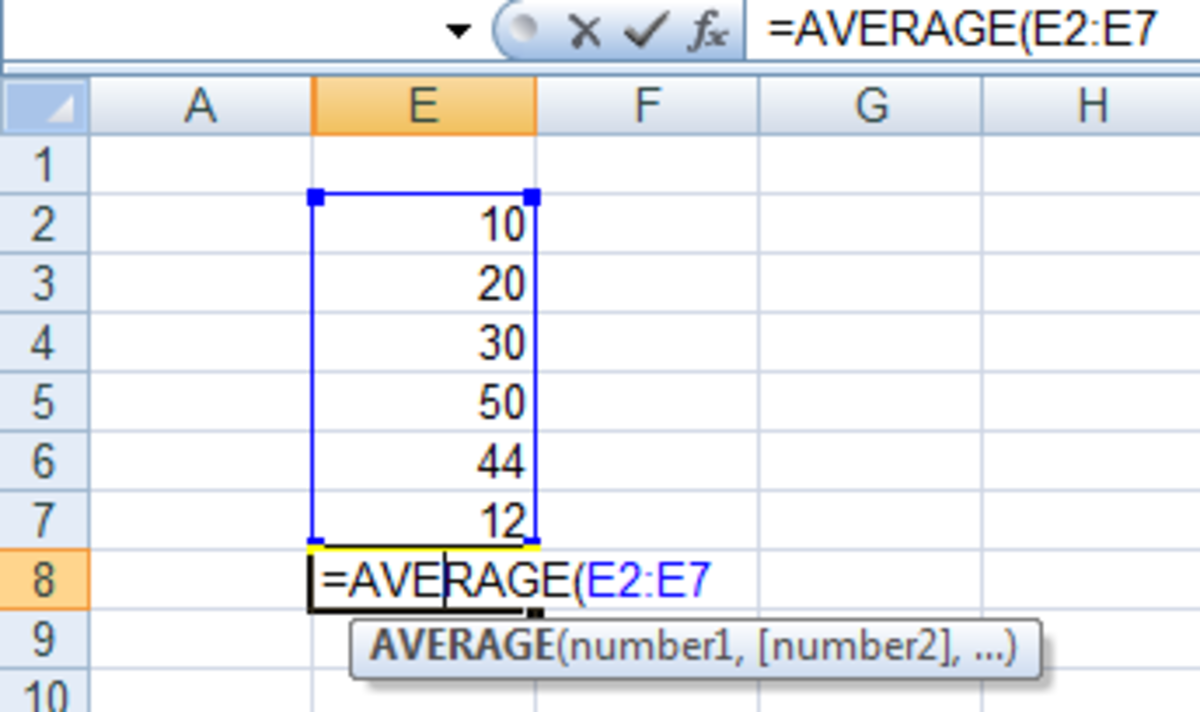How to use the AVERAGE function in a formula in Excel 2007 and Excel 2010.http://robbiecwilson.hubpages.com/

The final function we will look at is COUNT. This function counts the number of cells in the range that are not blank. In this example, the answer is five.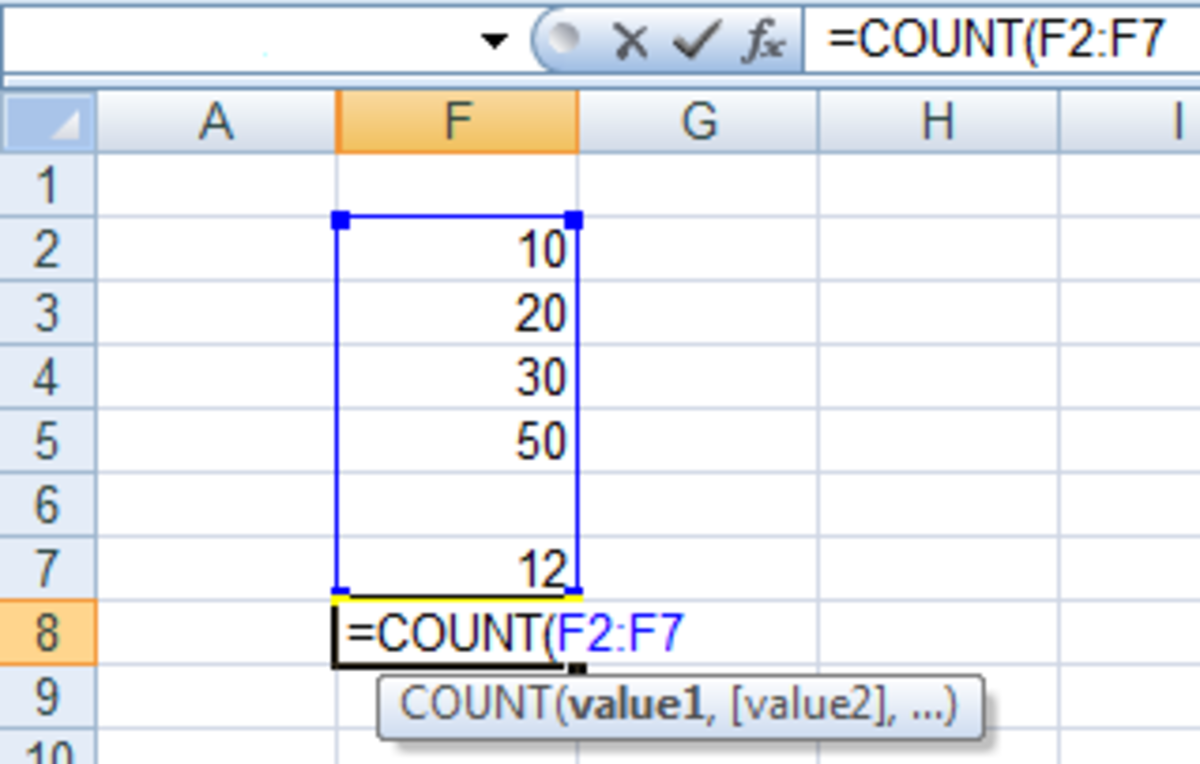How to use the COUNT function in a formula in Excel 2007 and Excel 2010.http://robbiecwilson.hubpages.com/

Both SUM and COUNT have variations that include an IF statement. So SUMIF will data if a certain criteria is met. So for example, I can use Excel to sum all sales of CDs.

COUNTIF works in a similar manner to SUMIF. It will count the number of times something occurs within a range. So I could tell how many CD sales I had in January by asking Excel to count the number of times CD occurs in my January sales data.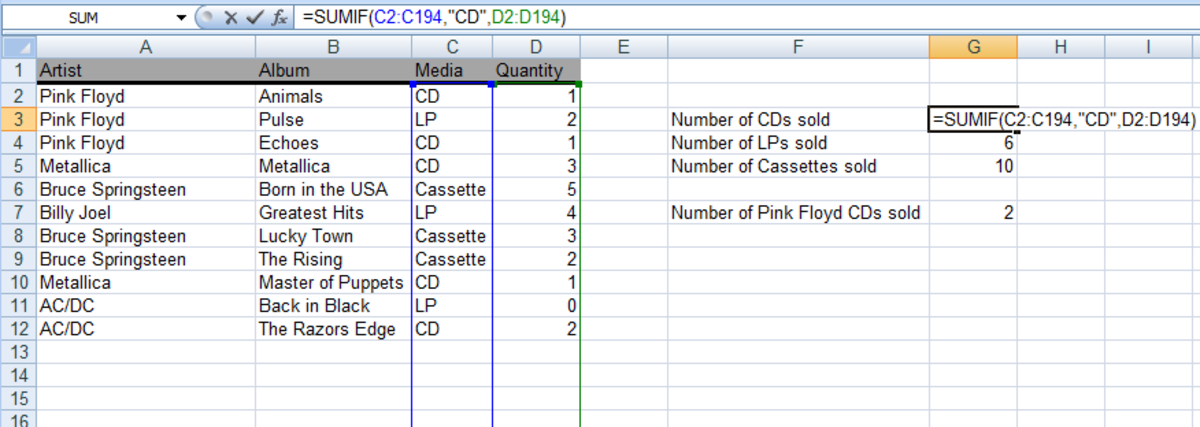Example of a formula using the SUMIF function in Excel 2007 and Excel 2010.http://robbiecwilson.hubpages.com/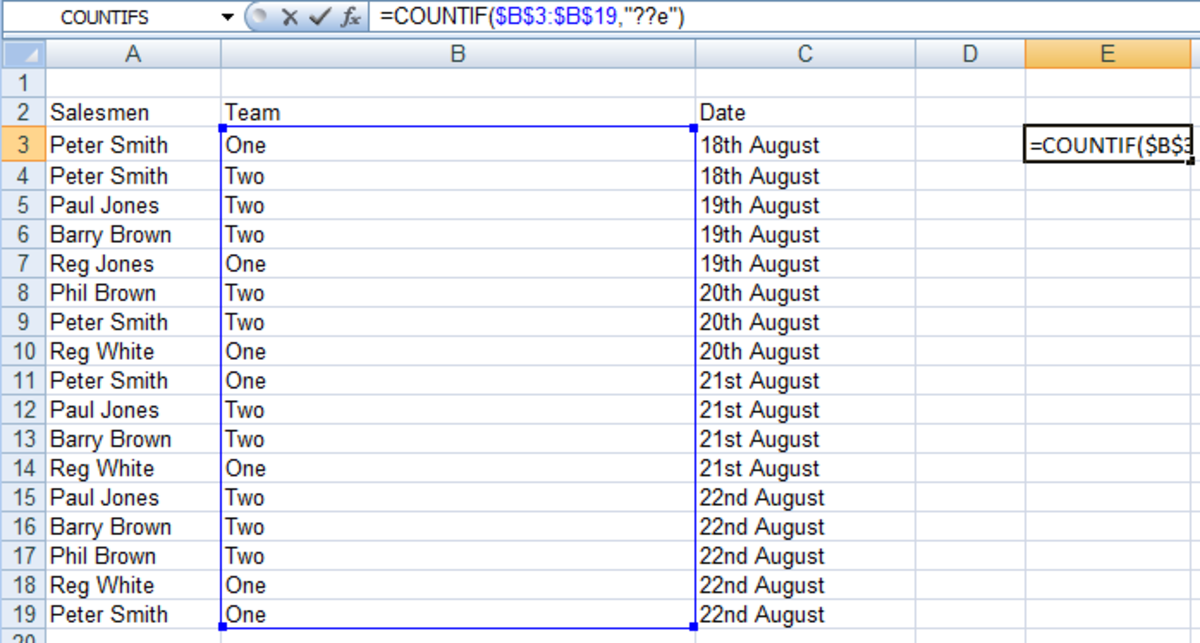How to use the COUNTIF function in a formula in Excel 2007 and Excel 2010.http://robbiecwilson.hubpages.com/

## Conclusion

Excel is a versatile and very powerful piece of software. Unfortunately, this power and versatility can mean that using Excel can become complicated. In today’s article, I have provided a beginners guide to:

• Creating basic formulas
• Using mathematical operators such as + - * and /
• Using the advanced operators & and \$ which allow you to join cells together and also make a cell reference absolute (it will not change when copied)
• Finally, I looked at three very useful functions, SUM, AVERAGE and COUNT

Many thanks for reading; I hope that you have found this article useful and informative. Feel free to leave any comments you may have below.